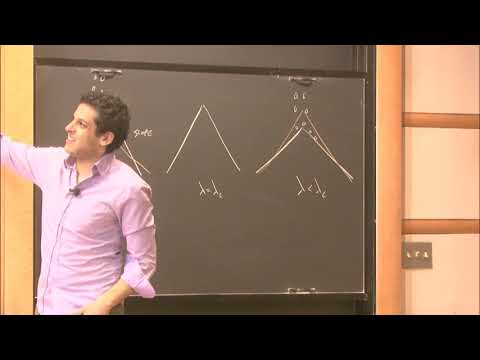### Videos

#### Halting problems for sandpiles and abelian networks

##### Presenter
March 12, 2019##### Abstract
Will this procedure be finite or infinite? If finite, how long can it last? Bjorner, Lovasz, and Shor asked these questions in 1991 about the following procedure, which goes by the name âabelian sandpileâ: Given a configuration of chips on the vertices of a finite directed graph, choose (however you like) a vertex with at least as many chips as out-neighbors, and send one chip from that vertex to each of its out-neighbors. Repeat, until there is no such vertex.Â The first part of the talk will be a little tour of âalgebraic directed graph theoryâ, whose main player is the graph Laplacian considered as an *integer* matrix. Iâll tell you about the curious class of coEulerian graphs, for which sandpile halting is easy to decide even though it may require exponentially many steps. A recent theorem of Nguyen and Wood, confirming a conjecture of Koplewitz, shows that coEulerian graphs are not rare: The directed random graph G(n,p) is coEulerian with limiting probability $1/(\zeta(2)\zeta(3)\zeta(4)\cdots )$ where $\zeta$ is the Riemann zeta function. So (in case you were wondering) about 43.6% of all simple directed graphs are coEulerian.Â The second part of the talk will focus on computation in abelian networks. These are automata networks, generalizing the abelian sandpile, for which the order of execution does not affect the final output. Iâll state some âlocal-to-global principlesâ of the form: If every automaton has property X, then the whole network has X.Â The usual Boolean gates are not abelian, but there is a set of simple "abelian logic gatesâ that suffice to compute any abelian function. These include an infinite family, indexed by prime numbers $p$, computing $x \mapsto \lfloor x/p \rfloor$. For directed acyclic circuits, no finite set of abelian gates suffices. But for circuits allowing a limited type of feedback, a specific set of five gates suffices.Â Is abelian computation weaker than Turing computation? If time permits, Iâll tell you what I know about this maddening question!Â If time still permits, Iâll return to the abelian sandpile to tell you about some of the âcritical exponentsâ physicists would like to calculate. Discrete Fourier techniques may be useful here.Â I will not answer any questions about hats.Â Joint work with Ben Bond, Matt Farrell, Alexander Holroyd, and Peter Winkler.